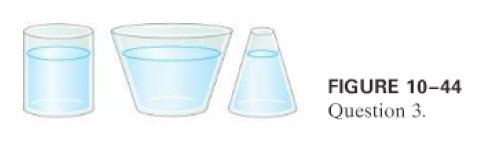### Solution Found!# The three containers in Fig. 10–44 are filled with water

Chapter 4, Problem 3Q

#### (choose chapter or problem)

Get Unlimited Answers! Check out our subscriptions

QUESTION:

The three containers in Fig.are filled with water to the same height and have the same surface area at the base; hence the water pressure, and the total force on the base of each, is the same. Yet the total weight of water is different for each. Explain this "hydrostatic paradox."Step 1 of 4

Letbe the mass of water in the first container. As a result, the downward force of gravity on the water is. Only a force perpendicular to the walls, horizontal for the first container, enters the side walls. A net upward force ofmust be applied to keep the water at rest. This is the normal force exerted by the container's bottom. This upward force equals the pressure at the container's bottom multiplied by the container's area, and it is the same for all three containers.

Become a subscriber and get unlimited answers!